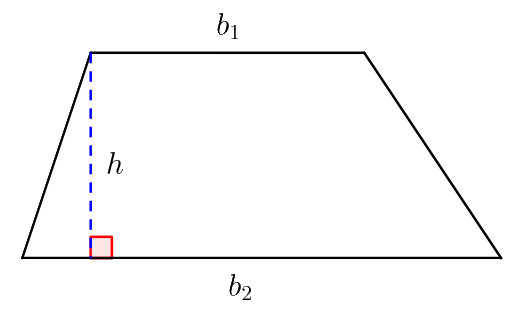231 views

The sides $\text{AB}$ and $\text{CD}$ of a trapezium $\text{ABCD}$ are parallel, with $\text{AB}$ being the smaller side. $\text{P}$ is the midpoint of $\text{CD}$ and $\text{ABPD}$ is a parallelogram. If the difference between the areas of the parallelogram $\text{ABPD}$ and the triangle $\text{BPC}$ is $10 \; \text{sq cm},$ then the area, $\text{in sq cm},$ of the trapezium $\text{ABCD}$  is

1. $25$
2. $30$
3. $40$
4. $20$

Let’s draw the trapezium for better understanding.Let $\text{PD} = a \; \text{cm} \Rightarrow \text{PC} = a \; \text{cm} \Rightarrow \text{CD} = 2a \; \text{cm}$

Area of the parallelogram $\text{ABPD}= a \times h \; \text{cm}^{2}$

Area of $\triangle \text{BPC} = \frac{1}{2} \times a \times h \; \text{cm}^{2}$

Now, $ah – \frac{1}{2}ah = 10$

$\Rightarrow 2ah – ah = 20$

$\Rightarrow \boxed{ah = 20 \; \text{cm}^{2}}$

$\therefore$ Area of the trapezium $\text{ABCD} = \frac{1}{2} (a + 2a) \times h$

$\qquad \qquad = \frac{1}{2} \times 3ah = \frac{1}{2} \times 3(20) = 30 \; \text{cm}^{2}.$

Correct Answer $: \text{B}$

$\textbf{PS:}$ A trapezium, also known as a trapezoid, is a quadrilateral in which a pair of sides are parallel, but the other pair of opposite sides are non-parallel. The area of a trapezium is computed with the following formula:

$$\text{Area}=\frac {1}{2} × \text {Sum of parallel sides} × \text{Distance between them}.$$The parallel sides are called the bases of the trapezium. Let $b_1$ and $b_2$​ be the lengths of these bases. The distance between the bases is called the height of the trapezium. Let $h$ be this height. Then this formula becomes:

$$\boxed{\text{Area}=\frac{1}{2}(b_1+b_2)h}$$

10.3k points

1 vote
1
180 views
1 vote